# PRESENTATION ON UNITIV SEMICONDUCTOR PHYSICS BY DR C

• Slides: 59PRESENTATION ON UNIT-IV SEMICONDUCTOR PHYSICS BY, DR. C. L. KHOBARAGADE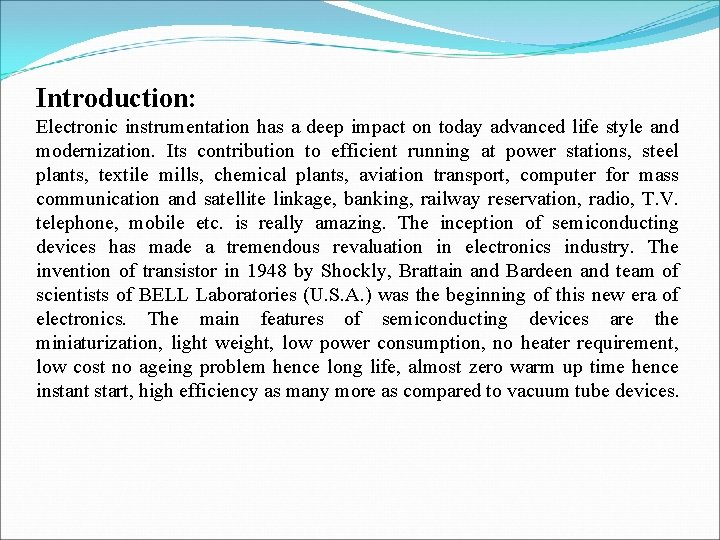Introduction: Electronic instrumentation has a deep impact on today advanced life style and modernization. Its contribution to efficient running at power stations, steel plants, textile mills, chemical plants, aviation transport, computer for mass communication and satellite linkage, banking, railway reservation, radio, T. V. telephone, mobile etc. is really amazing. The inception of semiconducting devices has made a tremendous revaluation in electronics industry. The invention of transistor in 1948 by Shockly, Brattain and Bardeen and team of scientists of BELL Laboratories (U. S. A. ) was the beginning of this new era of electronics. The main features of semiconducting devices are the miniaturization, light weight, low power consumption, no heater requirement, low cost no ageing problem hence long life, almost zero warm up time hence instant start, high efficiency as many more as compared to vacuum tube devices.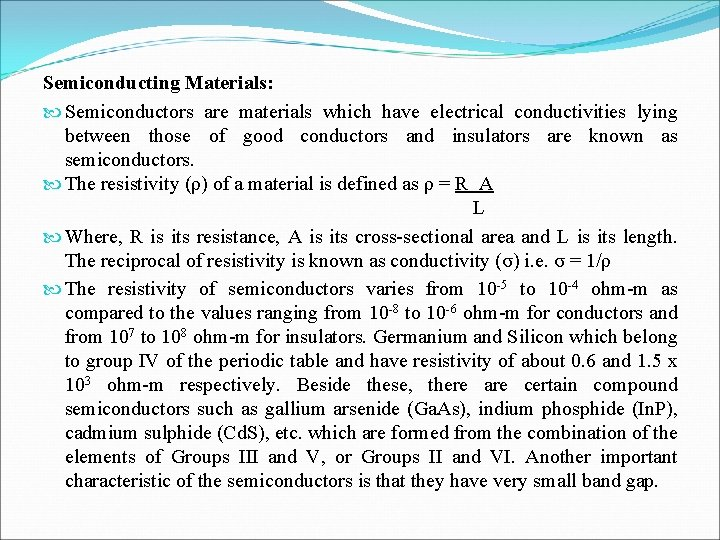Semiconducting Materials: Semiconductors are materials which have electrical conductivities lying between those of good conductors and insulators are known as semiconductors. The resistivity (ρ) of a material is defined as ρ = R A L Where, R is its resistance, A is its cross-sectional area and L is its length. The reciprocal of resistivity is known as conductivity (σ) i. e. σ = 1/ρ The resistivity of semiconductors varies from 10 -5 to 10 -4 ohm-m as compared to the values ranging from 10 -8 to 10 -6 ohm-m for conductors and from 107 to 108 ohm-m for insulators. Germanium and Silicon which belong to group IV of the periodic table and have resistivity of about 0. 6 and 1. 5 x 103 ohm-m respectively. Beside these, there are certain compound semiconductors such as gallium arsenide (Ga. As), indium phosphide (In. P), cadmium sulphide (Cd. S), etc. which are formed from the combination of the elements of Groups III and V, or Groups II and VI. Another important characteristic of the semiconductors is that they have very small band gap.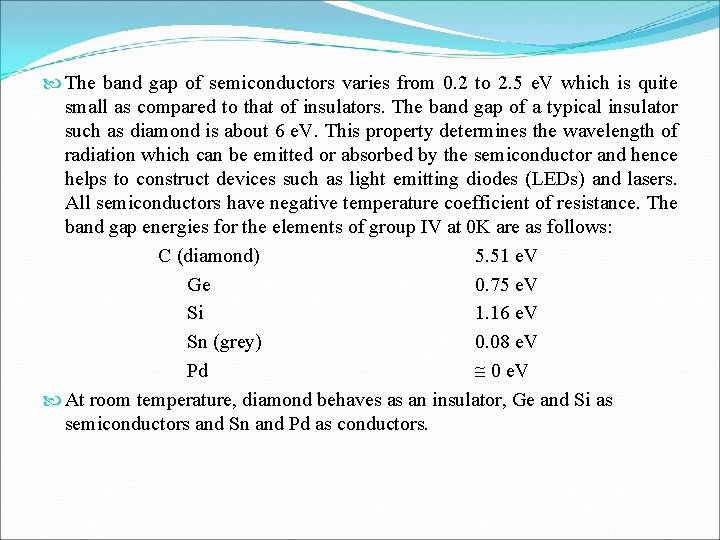The band gap of semiconductors varies from 0. 2 to 2. 5 e. V which is quite small as compared to that of insulators. The band gap of a typical insulator such as diamond is about 6 e. V. This property determines the wavelength of radiation which can be emitted or absorbed by the semiconductor and hence helps to construct devices such as light emitting diodes (LEDs) and lasers. All semiconductors have negative temperature coefficient of resistance. The band gap energies for the elements of group IV at 0 K are as follows: C (diamond) 5. 51 e. V Ge 0. 75 e. V Si 1. 16 e. V Sn (grey) 0. 08 e. V Pd 0 e. V At room temperature, diamond behaves as an insulator, Ge and Si as semiconductors and Sn and Pd as conductors.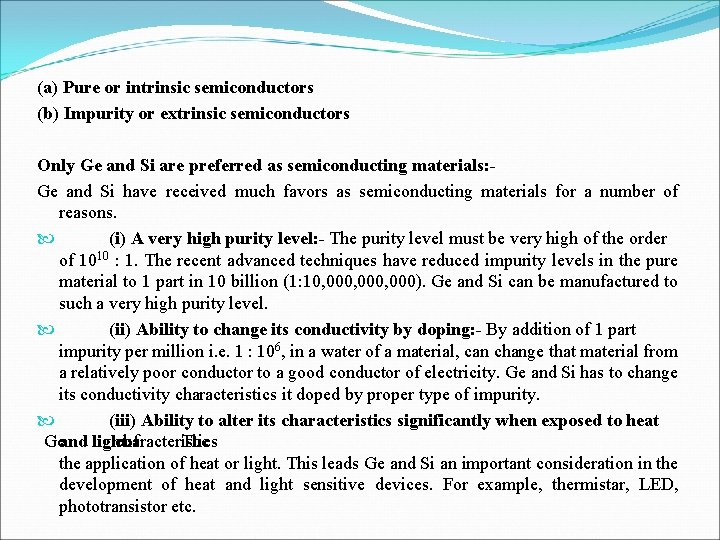(a) Pure or intrinsic semiconductors (b) Impurity or extrinsic semiconductors Only Ge and Si are preferred as semiconducting materials: - Ge and Si have received much favors as semiconducting materials for a number of reasons. (i) A very high purity level: - The purity level must be very high of the order of 1010 : 1. The recent advanced techniques have reduced impurity levels in the pure material to 1 part in 10 billion (1: 10, 000, 000). Ge and Si can be manufactured to such a very high purity level. (ii) Ability to change its conductivity by doping: - By addition of 1 part impurity per million i. e. 1 : 106, in a water of a material, can change that material from a relatively poor conductor to a good conductor of electricity. Ge and Si has to change its conductivity characteristics it doped by proper type of impurity. (iii) Ability to alter its characteristics significantly when exposed to heat Ge and light: characteristics of The the application of heat or light. This leads Ge and Si an important consideration in the development of heat and light sensitive devices. For example, thermistar, LED, phototransistor etc.Intrinsic semiconductor: - The intrinsic semiconductor are such as pure Ge or Si are undoped semiconductors. The electrical conductivity of this type of semiconductor is solely determined by thermally generated carriers. To understand the mechanism of conduction, we consider the bonding between atoms in these semicnoductors. Each having four valance electrons. Consider the case of Si. The atomic number is 14. The electronic configuration is (2, 8, 4) i. e. electrons in K-shell, 8 in L-shell and 4 in M-shell. Since Si has tetrahedral structure, each atom of silicon shares one electron each, with four neighbouring atoms by covalent bonds as shown in fig. 1. 1. At 0 K or of absolute zero all the four valence electrons are participated in covalent bonds, no free electron exists in the solid. Consequently, the semiconductor at 0 K cannot conduct and acts as a pure insulator.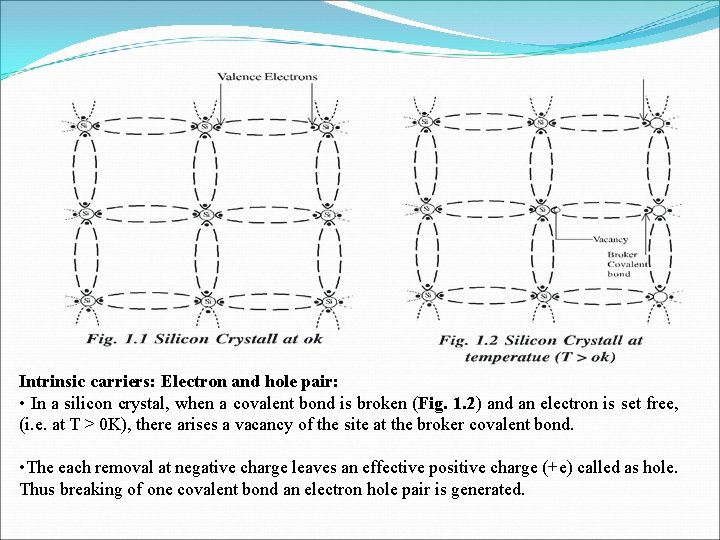Intrinsic carriers: Electron and hole pair: • In a silicon crystal, when a covalent bond is broken (Fig. 1. 2) and an electron is set free, (i. e. at T > 0 K), there arises a vacancy of the site at the broker covalent bond. • The each removal at negative charge leaves an effective positive charge (+e) called as hole. Thus breaking of one covalent bond an electron hole pair is generated.Recombination: - along with the generation of free electron hole pairs another process called `recombination `takes place. A free electron moving at random in a semiconductor crystal may encounter a hole and combine with it to construct a broken bond. Thus an electron hole pair disappears and the free electron is converted into bound electron. This recombination process takes place when the electron jumping from conduction band to valence band with release of energy (E = hν). In an intrinsic semiconductors when an equilibrium state is reached, the rate of generation of electron hole pairs (g) is equal to their recombination rate (r) i. e. g = r.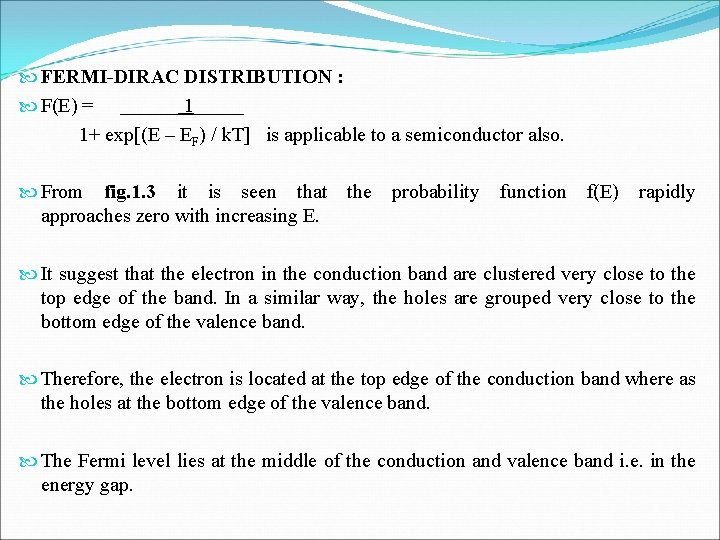FERMI-DIRAC DISTRIBUTION : F(E) = 1+ exp[(E – EF) / k. T] is applicable to a semiconductor also. From fig. 1. 3 it is seen that the probability function f(E) rapidly approaches zero with increasing E. It suggest that the electron in the conduction band are clustered very close to the top edge of the band. In a similar way, the holes are grouped very close to the bottom edge of the valence band. Therefore, the electron is located at the top edge of the conduction band where as the holes at the bottom edge of the valence band. The Fermi level lies at the middle of the conduction and valence band i. e. in the energy gap.Effect of temperature on Fermi Level: -Variation of Fermi level with temperature in an intrinsic semiconductor is shown in (Fig. 1. 4). The variation is mainly due to the changes of effective masses of electron (me) and hole (mh) with temperature. As the temperature increases the Fermi level gets displaced towards the bottom of the conduction band if mh > me and gets displaced towards the top edge of the valence band if mh < me for most of the materials, the shift of Fermi level is negligible due to mh = me. Electron and Hole Concentration: - In intrinsic semiconductors the charge carriers are nothing but electrons in the conduction band holes in the valence band. Since these carriers are generated due to the breaking of covalent bands, we have the equal number of electrons and holes i. e. at ok, the semiconductor act as an insulator. With the increase of thermal energy the covalent bonds are broken and electron hole pairs are created.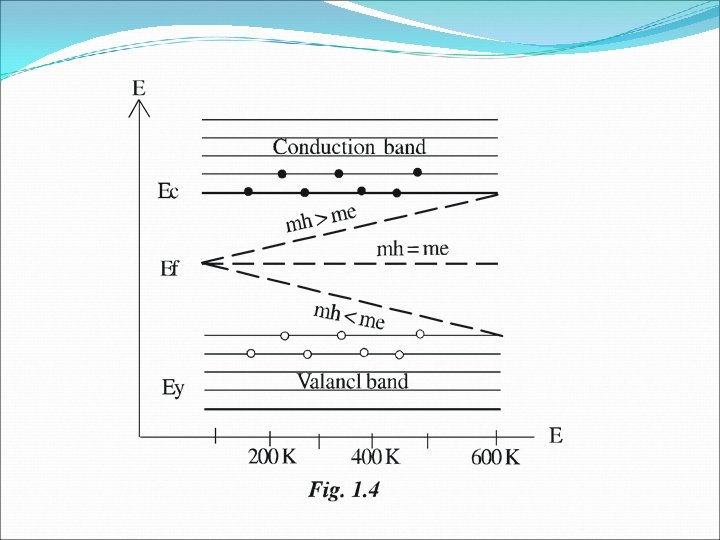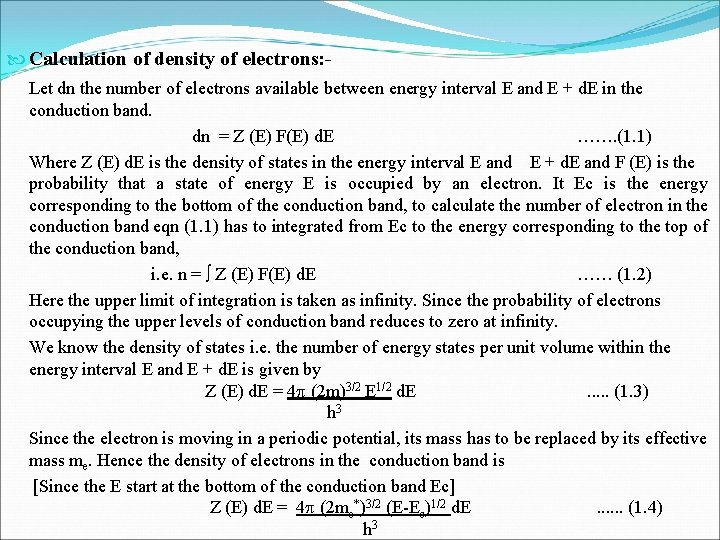Calculation of density of electrons: - Let dn the number of electrons available between energy interval E and E + d. E in the conduction band. dn = Z (E) F(E) d. E ……. (1. 1) Where Z (E) d. E is the density of states in the energy interval E and E + d. E and F (E) is the probability that a state of energy E is occupied by an electron. It Ec is the energy corresponding to the bottom of the conduction band, to calculate the number of electron in the conduction band eqn (1. 1) has to integrated from Ec to the energy corresponding to the top of the conduction band, i. e. n = Z (E) F(E) d. E …… (1. 2) Here the upper limit of integration is taken as infinity. Since the probability of electrons occupying the upper levels of conduction band reduces to zero at infinity. We know the density of states i. e. the number of energy states per unit volume within the energy interval E and E + d. E is given by Z (E) d. E = 4 (2 m)3/2 E 1/2 d. E . . . (1. 3) h 3 Since the electron is moving in a periodic potential, its mass has to be replaced by its effective mass me. Hence the density of electrons in the conduction band is [Since the E start at the bottom of the conduction band Ec] Z (E) d. E = 4 (2 me*)3/2 (E-Ec)1/2 d. E . . . (1. 4) h 3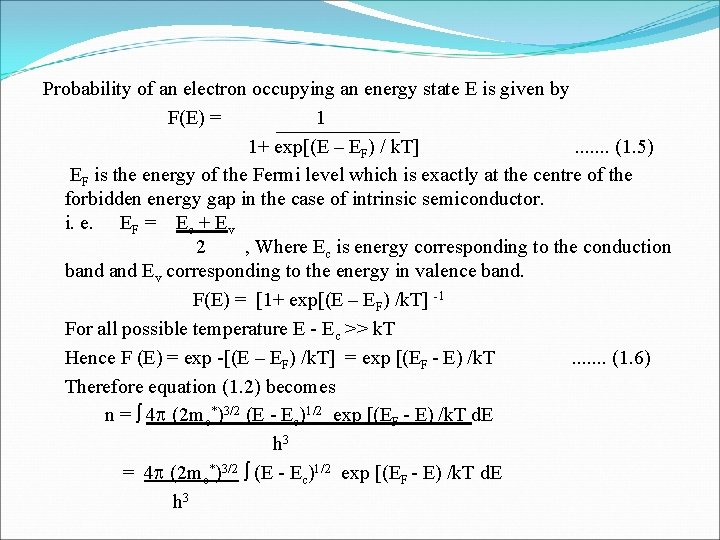Probability of an electron occupying an energy state E is given by F(E) = 1 1+ exp[(E – EF) / k. T] . . . . (1. 5) EF is the energy of the Fermi level which is exactly at the centre of the forbidden energy gap in the case of intrinsic semiconductor. i. e. EF = Ec + Ev 2 , Where Ec is energy corresponding to the conduction band Ev corresponding to the energy in valence band. F(E) = [1+ exp[(E – EF) /k. T] -1 For all possible temperature E - Ec >> k. T Hence F (E) = exp -[(E – EF) /k. T] = exp [(EF - E) /k. T . . . . (1. 6) Therefore equation (1. 2) becomes n = 4 (2 me*)3/2 (E - Ec)1/2 exp [(EF - E) /k. T d. E h 3 = 4 (2 me*)3/2 (E - Ec)1/2 exp [(EF - E) /k. T d. E h 3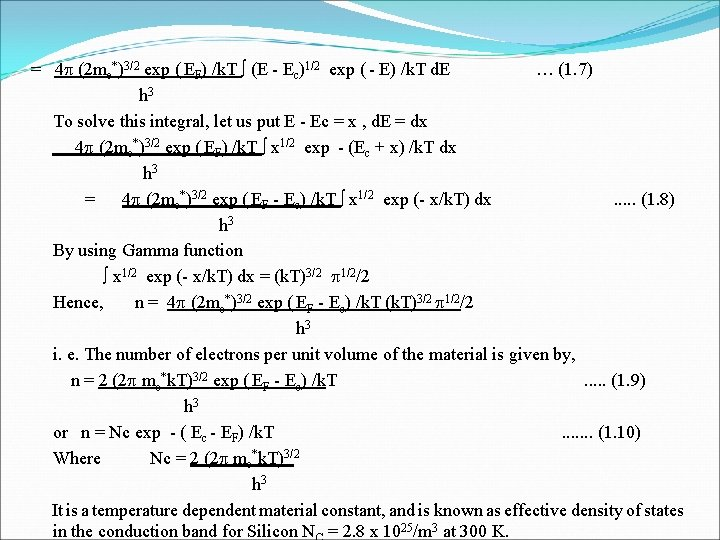= 4 (2 me*)3/2 exp ( EF) /k. T (E - Ec)1/2 exp ( - E) /k. T d. E … (1. 7) h 3 To solve this integral, let us put E - Ec = x , d. E = dx 4 (2 me*)3/2 exp ( EF) /k. T x 1/2 exp - (Ec + x) /k. T dx h 3 = 4 (2 me*)3/2 exp ( EF - Ec) /k. T x 1/2 exp (- x/k. T) dx . . . (1. 8) h 3 By using Gamma function x 1/2 exp (- x/k. T) dx = (k. T)3/2 1/2/2 Hence, n = 4 (2 me*)3/2 exp ( EF - Ec) /k. T (k. T)3/2 1/2/2 h 3 i. e. The number of electrons per unit volume of the material is given by, n = 2 (2 me*k. T)3/2 exp ( EF - Ec) /k. T . . . (1. 9) h 3 or n = Nc exp - ( Ec - EF) /k. T . . . . (1. 10) Where Nc = 2 (2 me*k. T)3/2 h 3 It is a temperature dependent material constant, and is known as effective density of states in the conduction band for Silicon N = 2. 8 x 1025/m 3 at 300 K.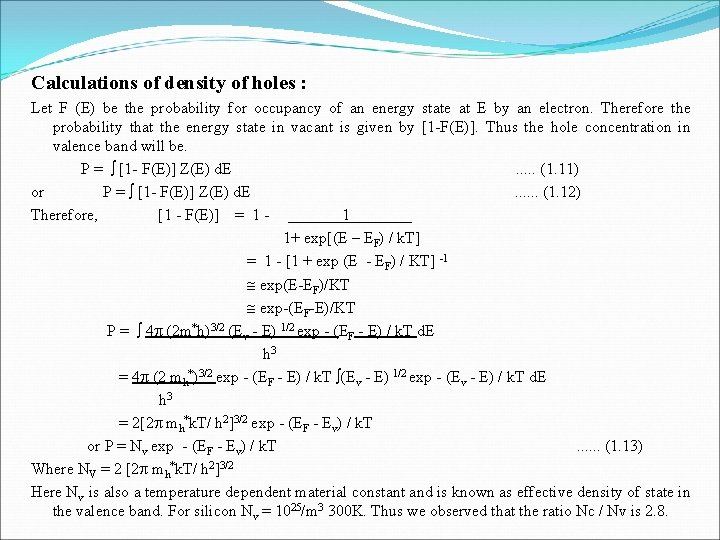Calculations of density of holes : Let F (E) be the probability for occupancy of an energy state at E by an electron. Therefore the probability that the energy state in vacant is given by [1 -F(E)]. Thus the hole concentration in valence band will be. P = [1 - F(E)] Z(E) d. E . . . (1. 11) or P = [1 - F(E)] Z(E) d. E . . . (1. 12) Therefore, [1 - F(E)] = 1 - 1 1+ exp[(E – EF) / k. T] = 1 - [1 + exp (E - EF) / KT] -1 exp(E-EF)/KT exp-(EF-E)/KT P = 4 (2 m*h)3/2 (Ev - E) 1/2 exp - (EF - E) / k. T d. E h 3 = 4 (2 mh*)3/2 exp - (EF - E) / k. T (Ev - E) 1/2 exp - (Ev - E) / k. T d. E h 3 = 2[2 mh*k. T/ h 2]3/2 exp - (EF - Ev) / k. T or P = Nv exp - (EF - Ev) / k. T . . . (1. 13) Where NV = 2 [2 mh*k. T/ h 2]3/2 Here Nv is also a temperature dependent material constant and is known as effective density of state in the valence band. For silicon Nv = 1025/m 3 300 K. Thus we observed that the ratio Nc / Nv is 2. 8.Intrinsic carrier concentration: - In intrinsic semiconductors, n = p. Hence n = p = ni is called intrinsic carrier concentration. Therefore, ni 2= np = 4 [2 k. T/ h 2]3/2 (me*mh*)3/2 exp - (Ev - Ec) / k. T = 4 [2 k. T/ h 2]3/2 (me*mh*)3/2 exp (- Eg / k. T) . . . (1. 14) Where EC - Ev = Eg is the forbidden energy gap. Hence, ni = 2 [2 k. T/ h 2]3/2 (me*mh*)3/4 exp (- Eg / 2 k. T) …. . (1. 15)Calculations for Fermi Level : Since n = p in intrinsic semiconductors. 2[2 me* k. T/ h 2]3/2 exp (EF - Ec)/k. T = 2[2 mh* k. T/ h 2]3/2 exp (Ev - EF)/k. T or (me*) 3/2 exp (EF - Ec)/k. T = (mh*)3/2 exp (Ev - EF)/k. T exp (2 EF)/k. T = (mh*/ me*)3/2 exp (Ev + Ec)/k. T Taking logarithms on both sides, we get 2 EF / k. T = 3/2 log mh*/ me* + (Ev + Ec) / k. T i. e. EF = (3 k. T/4) log mh*/ me* + (Ev + Ec) / 2 . . . (1. 16) If we assume that mh* = me* EF = (Ev + Ec) / 2 . . . (1. 17) Thus Fermi level is located half way between the valence band conduction bond and its position is independent of temperature. Since mh is greater than me, EF is just above the middle and rises slightly with increasing temperature shown in fig. 1. 4.Impurity or Extrinsic semiconductor (Doping) : A semiconducting material in which the charge carriers originate from impurity atoms added to the material is called impurity semiconductor or extrinsic semiconductor. The intrinsic semicnoductors, as such, are of little importance owing to their very small and fixed conductivity. (i) Donor or N-type semiconductor: The impurity atoms of group V are known as `donor’ or N-type and gives rise to n-type semiconductor. The Pentavalent elements such as phosphorous (p), (As) Arsenic or antimony (sb) have five electrons in their outermost orbits, The fifth electron is weekly bound to the parent atom. Even for lesser thermal energy this fifth electron is released leaving the parent atom positively ionized. During this process no hole generated. Since these pentavalent elements donate negatives charges (electrons) they are called N-type impurities and the semiconductors doped with pentavalent impurities are called N-type semiconductors.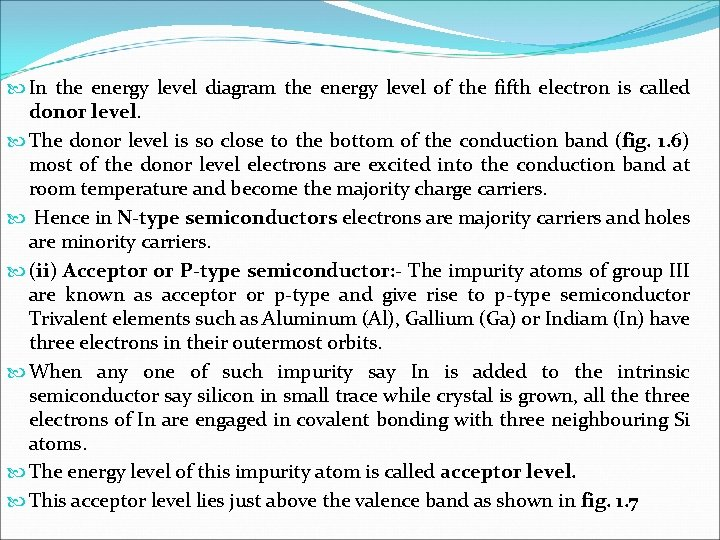In the energy level diagram the energy level of the fifth electron is called donor level. The donor level is so close to the bottom of the conduction band (fig. 1. 6) most of the donor level electrons are excited into the conduction band at room temperature and become the majority charge carriers. Hence in N-type semiconductors electrons are majority carriers and holes are minority carriers. (ii) Acceptor or P-type semiconductor: - The impurity atoms of group III are known as acceptor or p-type and give rise to p-type semiconductor Trivalent elements such as Aluminum (Al), Gallium (Ga) or Indiam (In) have three electrons in their outermost orbits. When any one of such impurity say In is added to the intrinsic semiconductor say silicon in small trace while crystal is grown, all the three electrons of In are engaged in covalent bonding with three neighbouring Si atoms. The energy level of this impurity atom is called acceptor level. This acceptor level lies just above the valence band as shown in fig. 1. 7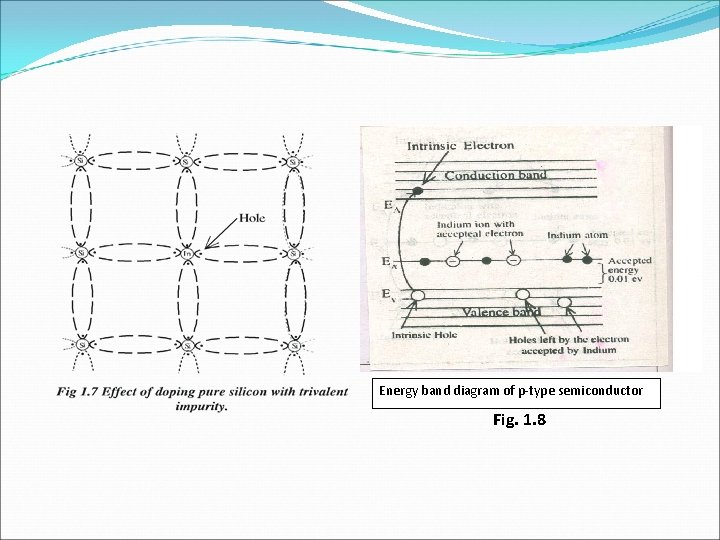Energy band diagram of p-type semiconductor Fig. 1. 8Hall Effect: - It a semiconductor carrying a current is placed in a magnetic field acting perpendicular to the direction of current then a potential difference is developed in a direction perpendicular to both, the current as well as magnetic field. This phenomenon is known as Hall Effect. This phenomenon discovered by the physicist E. H. Hall in 1879. The importance of Hall Effect (i) To determine the sign of charge carriers, (ii) To determine the charge concentration and. (iii) To determine the carrier mobility (iv) To determine the conductivity of the material of specimen. (v) To determine the Hall voltage. To determine the mobility of charge carriers if conductivity of the material is known.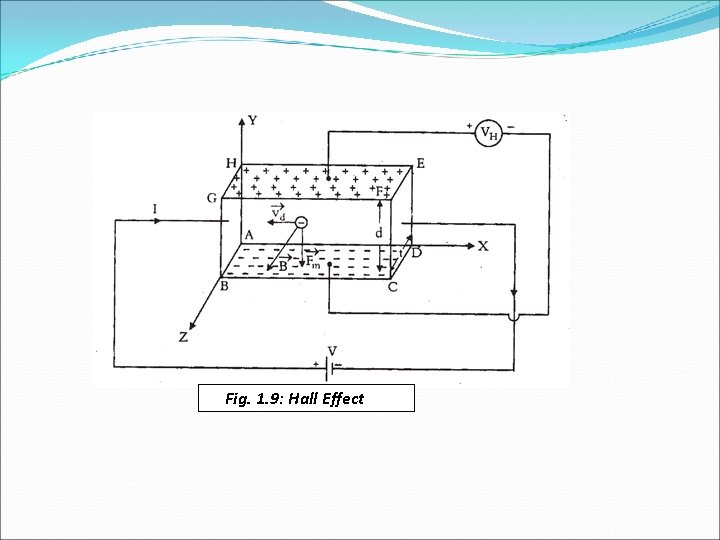Fig. 1. 9: Hall Effect(i) Negative charge carriers (N-type semiconductor) Let us consider n-type semiconductor ABCDEFGH carrying a current I in the positive x-direction. A magnetic field B is applied to the plate in the positive Z - direction, then B = Bkˆ. Since material is n-types, the charge carriers are electrons. As the current is flowing in + x-direction, the free electrons are drifting in the - x direction. If vd is the drift velocity of electrons, then magnetic force Fm on each electron Fm is given by Fm = - e vd x B = e (- vd i x Bkˆ) = - e vd B j . . . (1. 40) The force Fm acts in the -ve direction. This result the electrons to bend downward towards the face ABCD. The electrons accumulate on the lower surface producing a net negative charge on face ABCD. As a result a net positive charge is developed on the upper surface EFGH.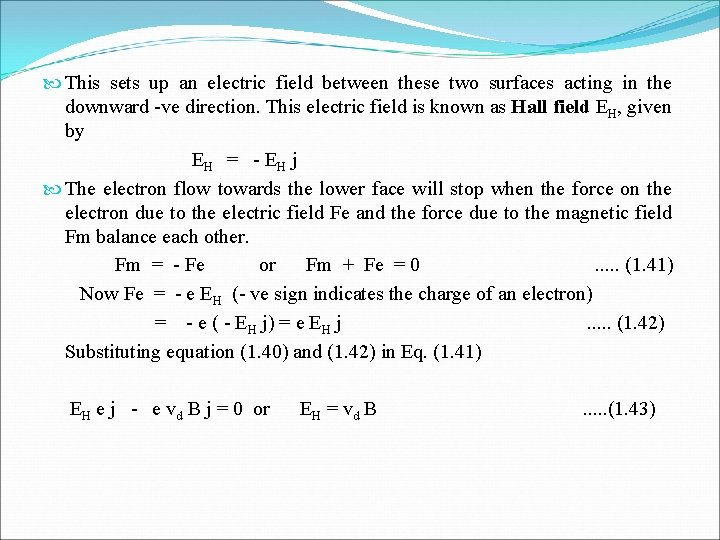This sets up an electric field between these two surfaces acting in the downward -ve direction. This electric field is known as Hall field EH, given by EH = - EH j The electron flow towards the lower face will stop when the force on the electron due to the electric field Fe and the force due to the magnetic field Fm balance each other. Fm = - Fe or Fm + Fe = 0 . . . (1. 41) Now Fe = - e EH (- ve sign indicates the charge of an electron) = - e ( - EH j) = e EH j . . . (1. 42) Substituting equation (1. 40) and (1. 42) in Eq. (1. 41) EH e j - e vd B j = 0 or EH = vd B . . . (1. 43)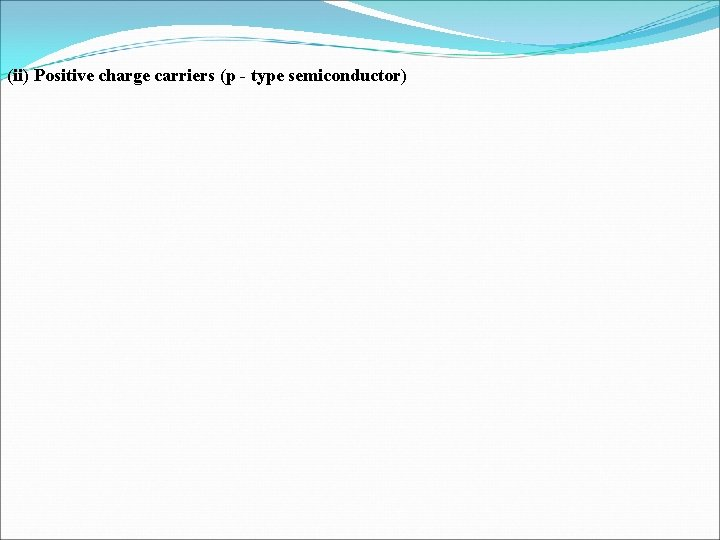(ii) Positive charge carriers (p - type semiconductor)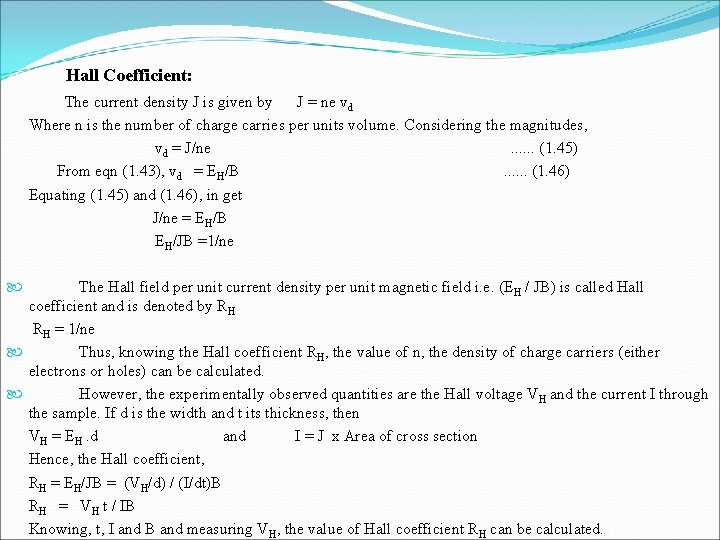Hall Coefficient: The current density J is given by J = ne vd Where n is the number of charge carries per units volume. Considering the magnitudes, vd = J/ne . . . (1. 45) From eqn (1. 43), vd = EH/B . . . (1. 46) Equating (1. 45) and (1. 46), in get J/ne = EH/B EH/JB =1/ne The Hall field per unit current density per unit magnetic field i. e. (EH / JB) is called Hall coefficient and is denoted by RH = 1/ne Thus, knowing the Hall coefficient RH, the value of n, the density of charge carriers (either electrons or holes) can be calculated. However, the experimentally observed quantities are the Hall voltage VH and the current I through the sample. If d is the width and t its thickness, then VH = EH. d and I = J x Area of cross section Hence, the Hall coefficient, RH = EH/JB = (VH/d) / (I/dt)B RH = VH t / IB Knowing, t, I and B and measuring VH, the value of Hall coefficient RH can be calculated.• Hall voltage (VH): Let VH be the potential difference developed between the two faces ABCD and EFGH and d is thickness or the distance between these two faces, then, EH = VH /d = vd B or VH = vd d B This equation gives Hall - Voltage (VH). Mobility (μ): The conductivity of a semiconducting material is given by σ = ne μ Where μ is the mobility of the charge carries. But, ne = 1/ RH, from eq. (1. 45) on substitution we obtain. σ = μ / RH Mobility, μ = σ RH Knowing, the Hall coefficient RH and the conductivity (σ) of the sample, the mobility (μ) of charge carrier can be calculated.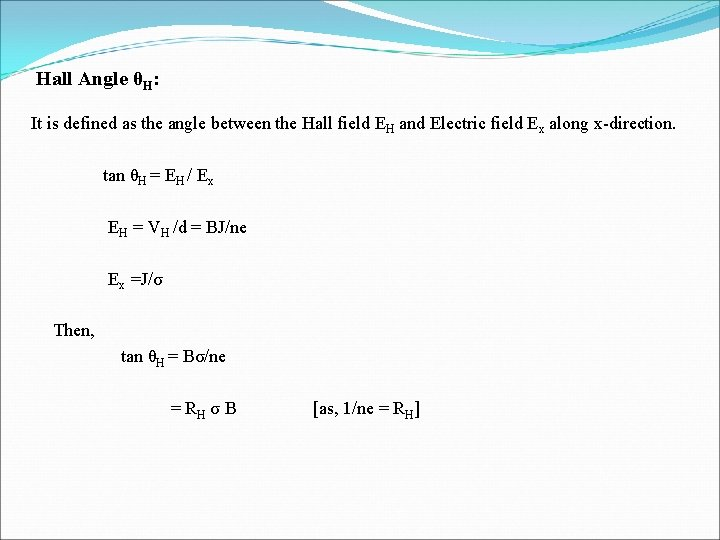Hall Angle θH: It is defined as the angle between the Hall field EH and Electric field Ex along x-direction. tan θH = EH / Ex EH = VH /d = BJ/ne Ex =J/σ Then, tan θH = Bσ/ne = RH σ B [as, 1/ne = RH]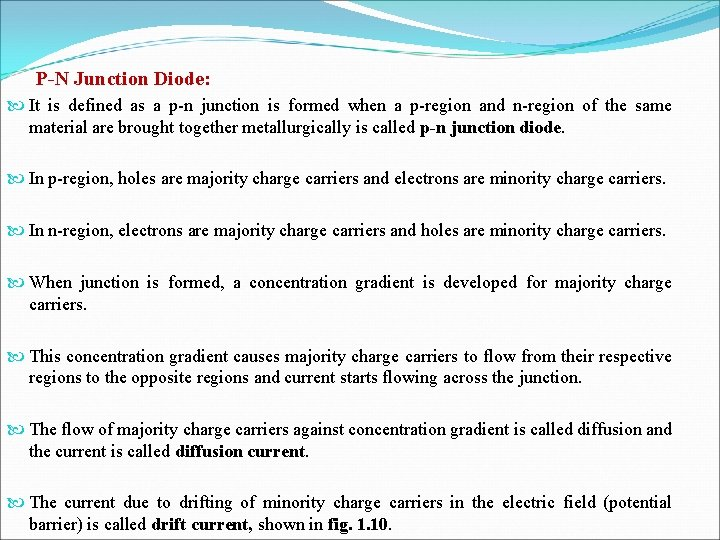P-N Junction Diode: It is defined as a p-n junction is formed when a p-region and n-region of the same material are brought together metallurgically is called p-n junction diode. In p-region, holes are majority charge carriers and electrons are minority charge carriers. In n-region, electrons are majority charge carriers and holes are minority charge carriers. When junction is formed, a concentration gradient is developed for majority charge carriers. This concentration gradient causes majority charge carriers to flow from their respective regions to the opposite regions and current starts flowing across the junction. The flow of majority charge carriers against concentration gradient is called diffusion and the current is called diffusion current. The current due to drifting of minority charge carriers in the electric field (potential barrier) is called drift current, shown in fig. 1. 10.Depletion Region: During the process, holes and electrons recombine near the junction, a region which is devoid of mobile charge carriers is formed near the junction. This region is called depletion region. When the holes and electrons recombine together, the charge neutrality condition of p and n-regions, separately gets disturbed. When a hole diffuses, small –ve charge is developed in p-region due to –vely charged acceptor impurity ions left behind. Similarly, when electron diffuses small +ve charge is developed due to +vely charged donor atoms left behind. Flow of charge carriers does not continue until all the carriers have recombined. Thus, a potential barrier is said to develop across the junction, shown in fig. 1. 11.fig. 1. 10 fig. 1. 11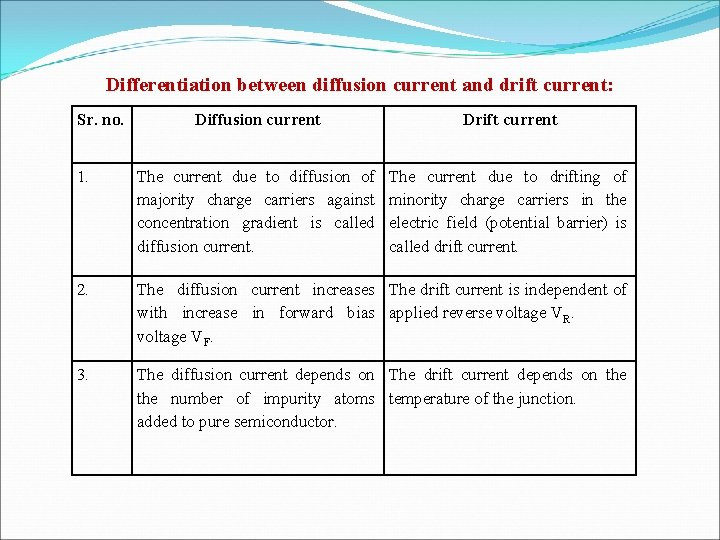Differentiation between diffusion current and drift current: Sr. no. Diffusion current Drift current 1. The current due to diffusion of majority charge carriers against concentration gradient is called diffusion current. The current due to drifting of minority charge carriers in the electric field (potential barrier) is called drift current. 2. The diffusion current increases The drift current is independent of with increase in forward bias applied reverse voltage VR. voltage VF. 3. The diffusion current depends on The drift current depends on the number of impurity atoms temperature of the junction. added to pure semiconductor.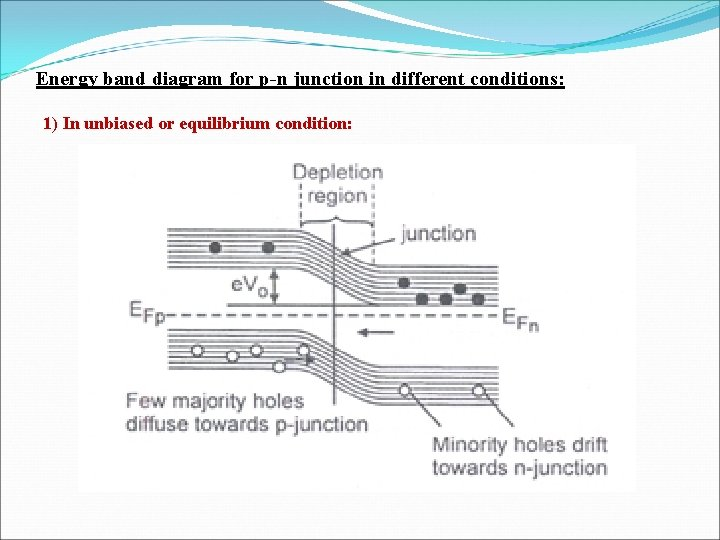Energy band diagram for p-n junction in different conditions: 1) In unbiased or equilibrium condition:2) In Forward biased condition by applying voltage (V) :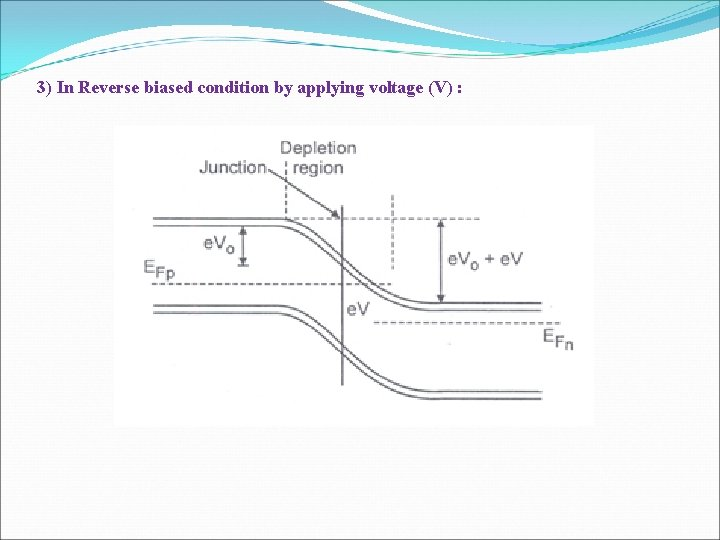3) In Reverse biased condition by applying voltage (V) :Biasing of p-n junction diode: Application of external voltage across the p-n junction so as to increase or decrease the barrier potential is called biasing. Two types of biasing: 1. Forward Biasing: The +ve terminal of supply is connected to p-terminal and –ve terminal of supply is connected to n-terminal of diode is called forward biasing. • Forward biasing causes the current to increase i. e. junction starts conducting where current flows from p to n-region. 2. Reverse Biasing: The +ve terminal of supply is connected to n-terminal and –ve terminal of supply is connected to p-terminal of diode is called reverse biasing. • Reverse biasing causes the current to decrease in diffusion current and the junction does not conduct practically. All above diagrams are shown below,Fig. 1. 12 Circuit symbol for p-n junction. Fig. 1. 13(a) Forward biased junction. Fig. 1. 13(b) reverse biased junction.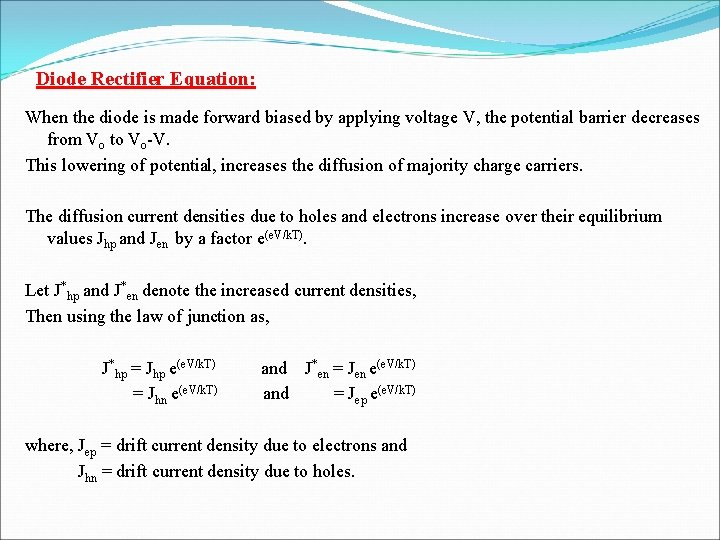Diode Rectifier Equation: When the diode is made forward biased by applying voltage V, the potential barrier decreases from Vo to Vo-V. This lowering of potential, increases the diffusion of majority charge carriers. The diffusion current densities due to holes and electrons increase over their equilibrium values Jhp and Jen by a factor e(e. V/k. T). Let J*hp and J*en denote the increased current densities, Then using the law of junction as, J*hp = Jhp e(e. V/k. T) and J*en = Jen e(e. V/k. T) = Jhn e(e. V/k. T) and = Jep e(e. V/k. T) where, Jep = drift current density due to electrons and Jhn = drift current density due to holes.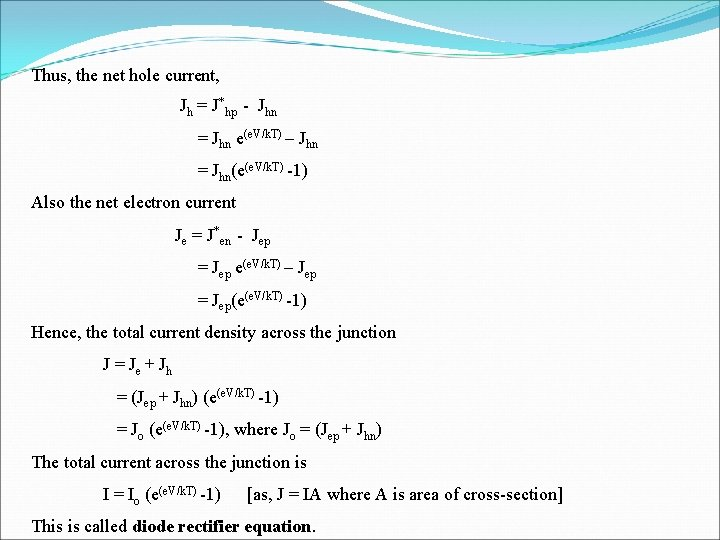Thus, the net hole current, Jh = J*hp - Jhn = Jhn e(e. V/k. T) – Jhn = Jhn(e(e. V/k. T) -1) Also the net electron current Je = J*en - Jep = Jep e(e. V/k. T) – Jep = Jep(e(e. V/k. T) -1) Hence, the total current density across the junction J = Je + Jh = (Jep + Jhn) (e(e. V/k. T) -1) = Jo (e(e. V/k. T) -1), where Jo = (Jep + Jhn) The total current across the junction is I = Io (e(e. V/k. T) -1) [as, J = IA where A is area of cross-section] This is called diode rectifier equation.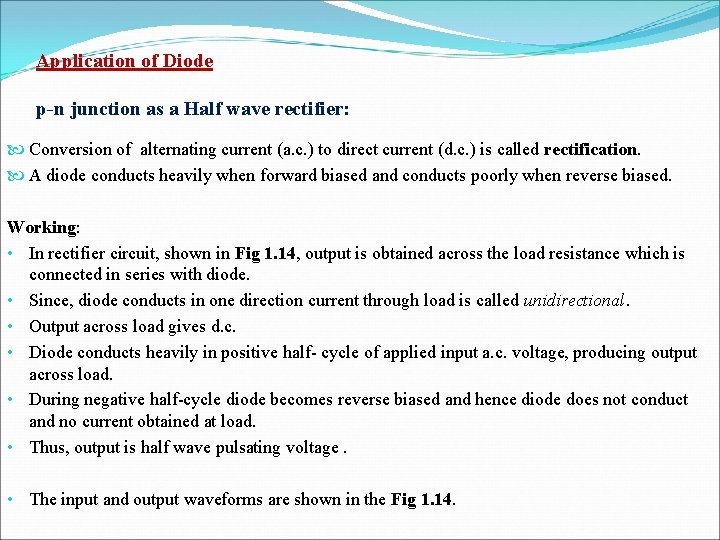Application of Diode p-n junction as a Half wave rectifier: Conversion of alternating current (a. c. ) to direct current (d. c. ) is called rectification. A diode conducts heavily when forward biased and conducts poorly when reverse biased. Working: • In rectifier circuit, shown in Fig 1. 14, output is obtained across the load resistance which is connected in series with diode. • Since, diode conducts in one direction current through load is called unidirectional. • Output across load gives d. c. • Diode conducts heavily in positive half- cycle of applied input a. c. voltage, producing output across load. • During negative half-cycle diode becomes reverse biased and hence diode does not conduct and no current obtained at load. • Thus, output is half wave pulsating voltage. • The input and output waveforms are shown in the Fig 1. 14.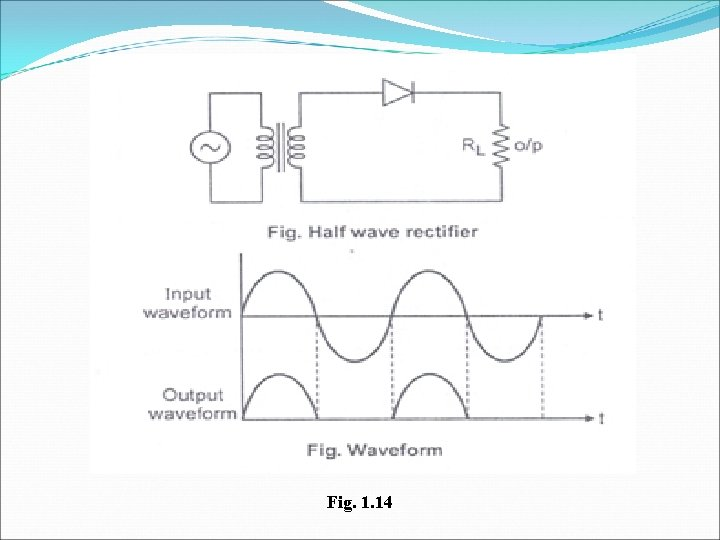Fig. 1. 14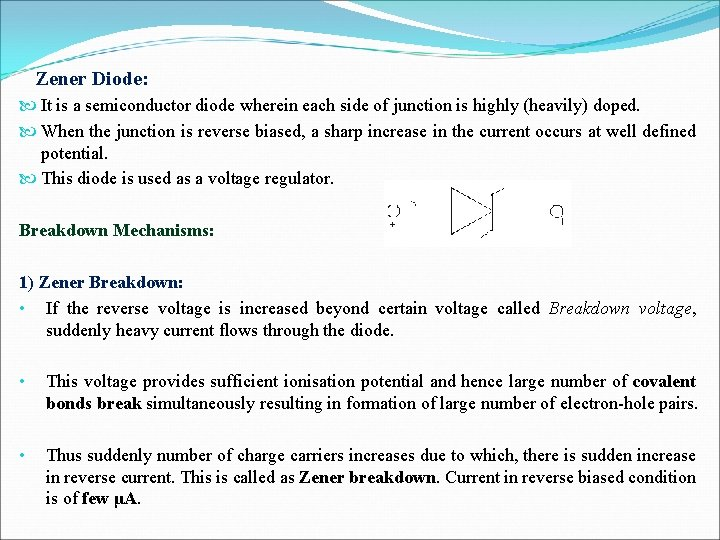Zener Diode: It is a semiconductor diode wherein each side of junction is highly (heavily) doped. When the junction is reverse biased, a sharp increase in the current occurs at well defined potential. This diode is used as a voltage regulator. Breakdown Mechanisms: 1) Zener Breakdown: • If the reverse voltage is increased beyond certain voltage called Breakdown voltage, suddenly heavy current flows through the diode. • This voltage provides sufficient ionisation potential and hence large number of covalent bonds break simultaneously resulting in formation of large number of electron-hole pairs. • Thus suddenly number of charge carriers increases due to which, there is sudden increase in reverse current. This is called as Zener breakdown. Current in reverse biased condition is of few μA.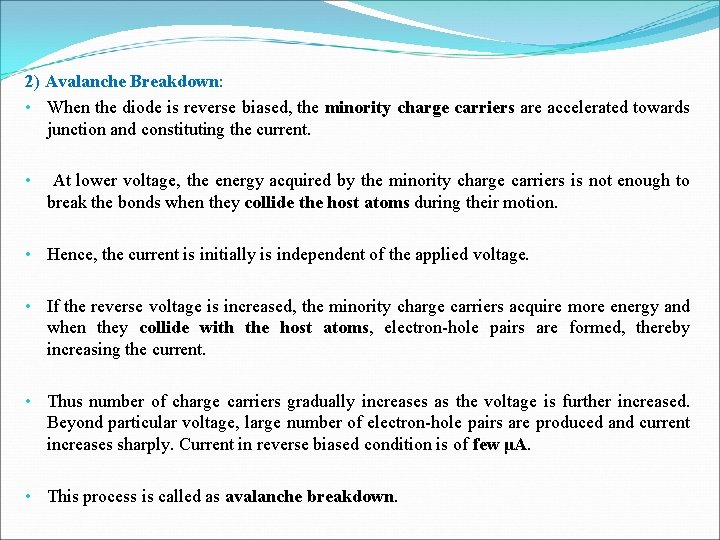2) Avalanche Breakdown: • When the diode is reverse biased, the minority charge carriers are accelerated towards junction and constituting the current. • At lower voltage, the energy acquired by the minority charge carriers is not enough to break the bonds when they collide the host atoms during their motion. • Hence, the current is initially is independent of the applied voltage. • If the reverse voltage is increased, the minority charge carriers acquire more energy and when they collide with the host atoms, electron-hole pairs are formed, thereby increasing the current. • Thus number of charge carriers gradually increases as the voltage is further increased. Beyond particular voltage, large number of electron-hole pairs are produced and current increases sharply. Current in reverse biased condition is of few μA. • This process is called as avalanche breakdown.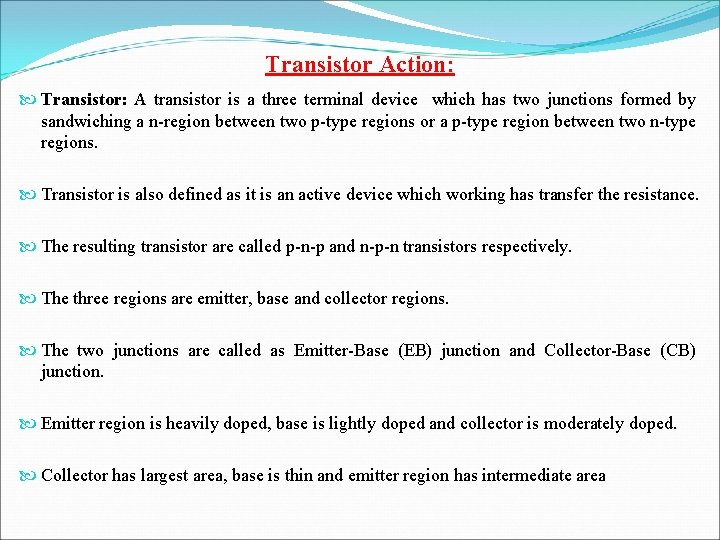Transistor Action: Transistor: A transistor is a three terminal device which has two junctions formed by sandwiching a n-region between two p-type regions or a p-type region between two n-type regions. Transistor is also defined as it is an active device which working has transfer the resistance. The resulting transistor are called p-n-p and n-p-n transistors respectively. The three regions are emitter, base and collector regions. The two junctions are called as Emitter-Base (EB) junction and Collector-Base (CB) junction. Emitter region is heavily doped, base is lightly doped and collector is moderately doped. Collector has largest area, base is thin and emitter region has intermediate area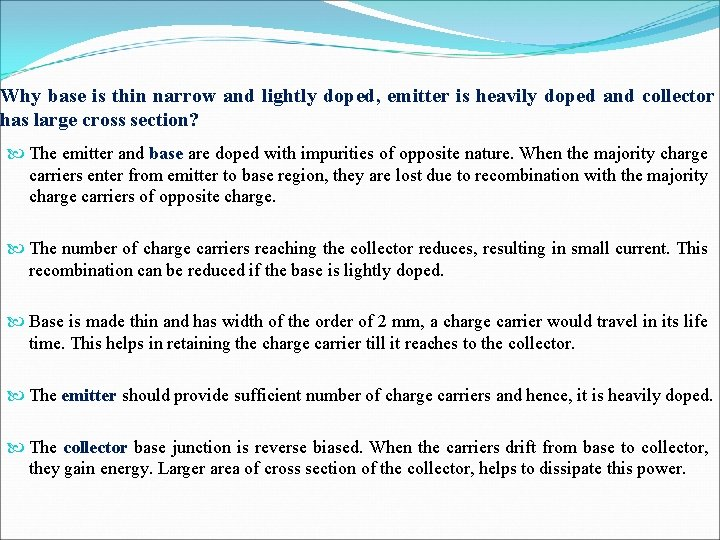Why base is thin narrow and lightly doped, emitter is heavily doped and collector has large cross section? The emitter and base are doped with impurities of opposite nature. When the majority charge carriers enter from emitter to base region, they are lost due to recombination with the majority charge carriers of opposite charge. The number of charge carriers reaching the collector reduces, resulting in small current. This recombination can be reduced if the base is lightly doped. Base is made thin and has width of the order of 2 mm, a charge carrier would travel in its life time. This helps in retaining the charge carrier till it reaches to the collector. The emitter should provide sufficient number of charge carriers and hence, it is heavily doped. The collector base junction is reverse biased. When the carriers drift from base to collector, they gain energy. Larger area of cross section of the collector, helps to dissipate this power.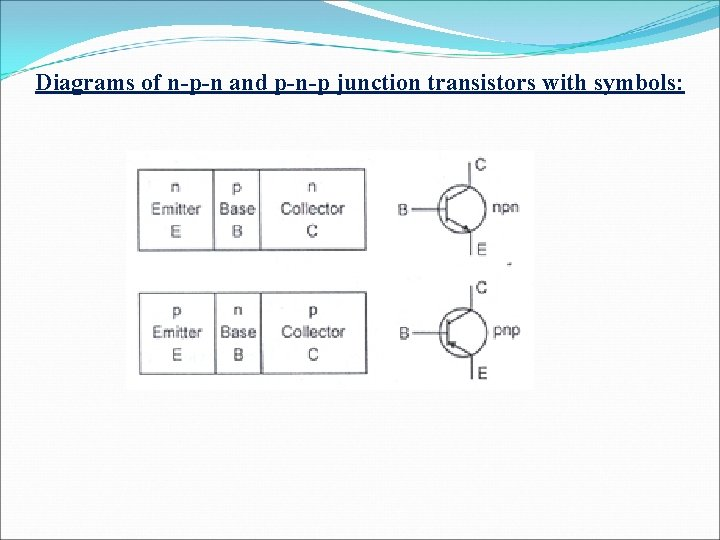Diagrams of n-p-n and p-n-p junction transistors with symbols: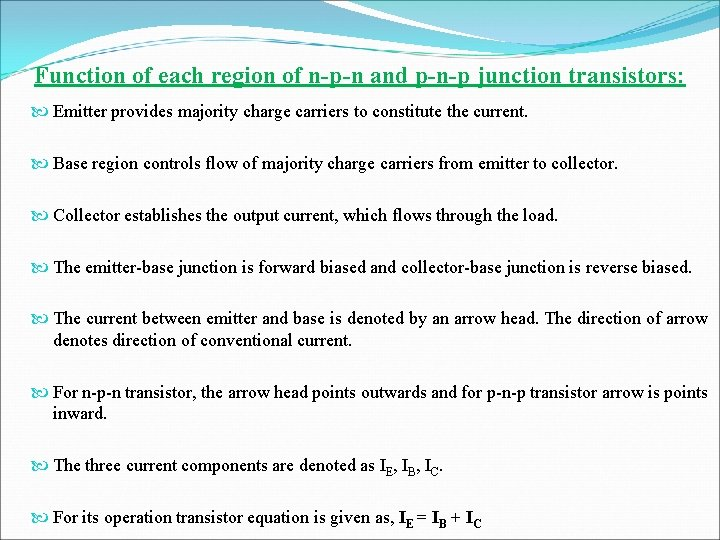Function of each region of n-p-n and p-n-p junction transistors: Emitter provides majority charge carriers to constitute the current. Base region controls flow of majority charge carriers from emitter to collector. Collector establishes the output current, which flows through the load. The emitter-base junction is forward biased and collector-base junction is reverse biased. The current between emitter and base is denoted by an arrow head. The direction of arrow denotes direction of conventional current. For n-p-n transistor, the arrow head points outwards and for p-n-p transistor arrow is points inward. The three current components are denoted as IE, IB, IC. For its operation transistor equation is given as, IE = IB + ICSymbols for n-p-n and p-n-p transistors along with current directions:Circuit diagram for n-p-n transistor in common base mode: In CB mode, base terminal is common to input and output circuit. For normal operation, the EB junction is forward biased and CB junction is made reverse biased. The sources VEB and VCB are used for this proper biasing.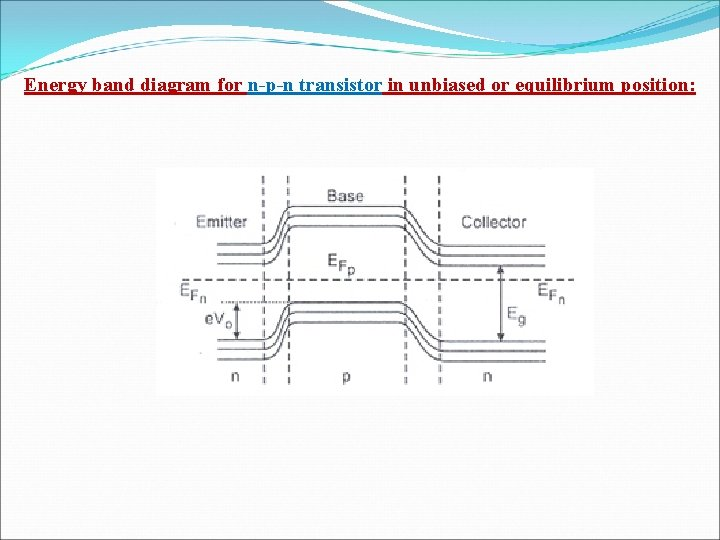Energy band diagram for n-p-n transistor in unbiased or equilibrium position: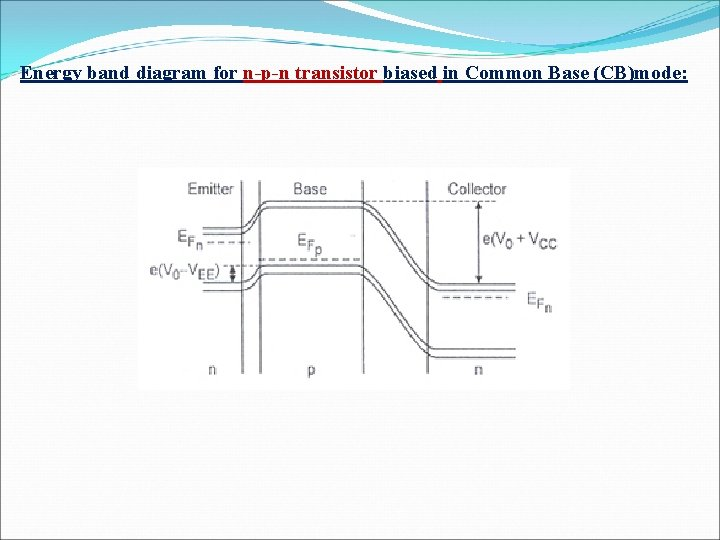Energy band diagram for n-p-n transistor biased in Common Base (CB)mode: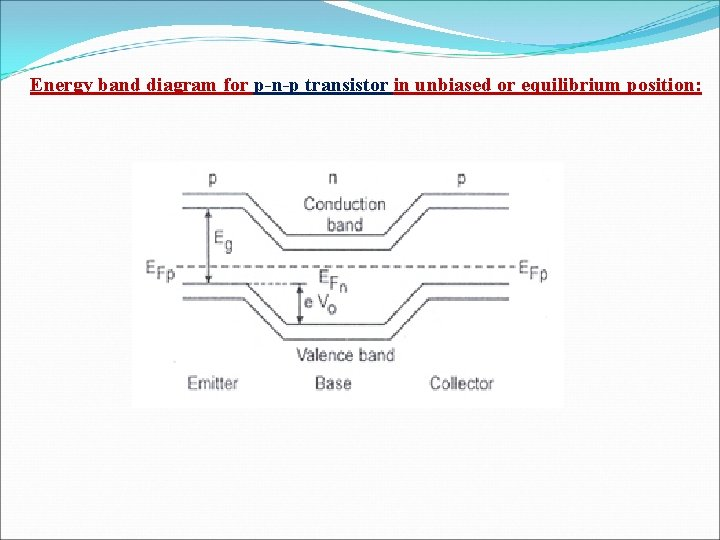Energy band diagram for p-n-p transistor in unbiased or equilibrium position: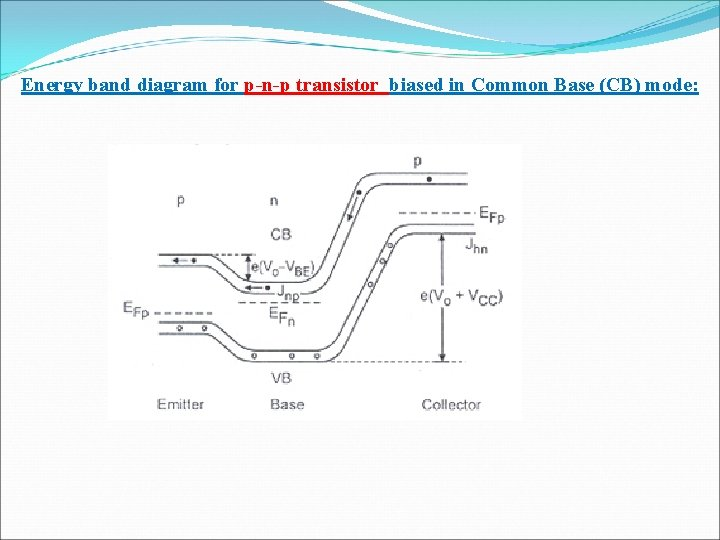Energy band diagram for p-n-p transistor biased in Common Base (CB) mode: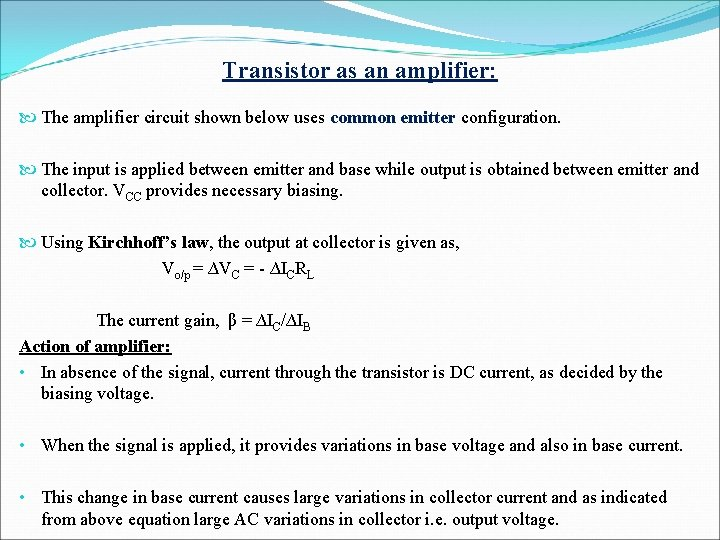Transistor as an amplifier: The amplifier circuit shown below uses common emitter configuration. The input is applied between emitter and base while output is obtained between emitter and collector. VCC provides necessary biasing. Using Kirchhoff’s law, the output at collector is given as, Vo/p = ∆VC = - ∆ICRL The current gain, β = ∆IC/∆IB Action of amplifier: • In absence of the signal, current through the transistor is DC current, as decided by the biasing voltage. • When the signal is applied, it provides variations in base voltage and also in base current. • This change in base current causes large variations in collector current and as indicated from above equation large AC variations in collector i. e. output voltage.Let, initially positive half-cycle appears at the input. This varying voltage increases the biasing of transistor increasing the base current and hence, collector current also increases. The variations in collector current produces variations in output voltage. Since the current gain β, is large, ∆IC >> ∆IB , the voltage at collector is amplified but is inverted. When the negative half-cycle appears at input, the biasing reduces, decreasing the base current, and hence collector current also decreases, producing variable output which is again amplified but inverted.Diagram for Transistor as an Amplifier:Any Questions? THANKS